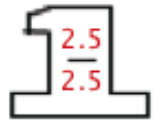### Home > MC1 > Chapter 7 > Lesson 7.1.2 > Problem7-21

7-21.

Use a Giant One to change each of the following fractions to a number written as a fraction over $100$. Then write each portion as a percent.

1.  $\frac { 3 } { 20 }$

$20\left(5\right) = 100$

1.  $\frac { 3 } { 40 }$

What do you multiply by $40$ to get $100$? Use the number in the Giant One.

$\frac{3}{40\ }\cdot$$=\frac{7.5}{100}=7.5$